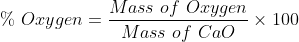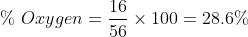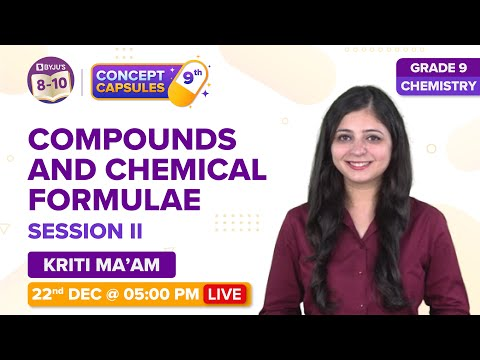Jet Set Go! All about Aeroplanes Jet Set Go! All about Aeroplanes

# Write the chemical formula of quick lime and slaked lime Questions

The chemical formula of a compound is a symbolic representation of its chemical composition. Chemical formulas reveal the elements that comprise a compound’s molecules, as well as the proportions in which the atoms of those elements combine to form those molecules. Quicklime and slaked lime have different proportions of atoms present in them.

 Definition: A chemical formula is a method of expressing information about the chemical quantities of atoms in a chemical compound or molecule using chemical element symbols, numbers, and other symbols such as dashes, parentheses, commas, brackets, and plus (+) and minus (-) signs.

## Write the chemical formula of quicklime and slaked lime questions Chemistry Questions with Solutions

Q-1: The chemical name of slaked lime is

a) Calcium oxide

b) Calcium hydroxide

c) Magnesium oxide

d) Magnesium hydroxide

Answer: b) Calcium hydroxide is the chemical name of slaked lime.

Q-2: What is another term for slaked lime?

a) Caustic lime

b) Lime water

c) Hydrated lime

d) All of the above

Answer: d) All of the above

Explanation: Calcium hydroxide, also known as caustic lime, hydrated lime, builders’ lime, slaked lime and pickling lime.

Q-3: CaO is the empirical formula for calcium oxide. Determine the oxygen percentage by mass in this compound. Atomic weights: Ca= 40 g/mol, O = 16 g/molQ-4: Which of the following is the correct chemical formula for quick lime?

a) Ca(OH)2

b) CaO

c) Na2O

d) NaOH

Explanation: CaO is the correct chemical formula for quick lime.

Q-5: How many grams of hydrogen are present in 2 moles of slaked lime?

Answer: The chemical formula for slaked lime is Ca(OH)2.

1 mole of Ca(OH)2 contains 2 Hydrogen atoms

2 moles of Ca(OH)2 contains 4 Hydrogen atoms

1 atom of hydrogen has a mass of 1g.

Thus, 4 hydrogen atoms will have a mass of 4g.

Q-6: In the reaction

CaCO3 + heat → A + CO2

a) Predict A and the valency of atoms present in it.

b) Identify the name of A

a) The reaction is: CaCO3 + heat → CaO + CO2

Thus, A is CaO.

The charge on the atoms in the compound is referred to as valency. Calcium is in the +2 oxidation state (charge) and O is in the -2 oxidation state in the compound CaO. As a result, the valency of both atoms is 2.

b) The name of A is calcium oxide or quicklime.

Q-7: Match column I with column II

Column I

Column II

A) Milk of magnesia

i) NaOH

B) Slaked lime

ii) NaHCO3

C) Caustic Soda

iii) Ca(OH)2

D) Baking Soda

iv) CaO

E) Quick lime

v) Mg(OH)2

Answers: A)-v), B)-iii), C)-i), D)-ii) , E)-iv)

Q-8: How much slaked lime would be needed to completely decompose 8 grams of ammonium chloride?

Answer: The equation representing the decomposition of NH4Cl by slaked lime, that is, Ca(OH)2 is

Ca(OH)2 + 2NH4Cl → CaCl2 + 2NH3 + 2H2O

Molar mass of Ca(OH)2 = 74 g

Molar mass of NH4Cl = 107 g

From the above equation, we can see that

107 g of NH4Cl are decomposed by 74 g of Ca(OH)2

8 g of NH4Cl are decomposed by Ca(OH)2 = (8×74)/107 = 5.53 g

Q-9: Quick lime contains

a) only covalent bonds

b) only ionic bonds

c) one covalent and one ionic bond

d) one ionic and one coordinate bond

Explanation: In quick lime(CaO), Ca2+ ion is bonded to O2- via an ionic bond. It is an ionic compound.

Q-10: The Ca2+ ion constituent in quicklime’s chemical formula is isoelectronic with

a) Chlorine

b) Neon

c) Argon

d) Potassium

Explanation: There are 18 electrons in the Ca2+ ion. Argon is the only element in here with 18 electrons. As both have the same number of electrons,therefore they are isoelectronic species.

Q-11: Write the atomicity of different constituent ions in slaked lime’s chemical formula.

Answer: Slaked lime contains the constituent ions OH and Ca2+. Ca2+ is a monatomic cation because it only has one atom, whereas OH is a diatomic anion because it has two atoms- oxygen and hydrogen.

Q-12: The chemical formula for slaked lime is Ca(OH)2. How many electrovalent bonds does it contain?

Answer: Ca+2 ion form one ionic bond with each OH ion in slaked lime. Thus it contains two electrovalent bonds(ionic bonds).

Q-13: When one mole of calcium phosphide reacts with an excess of water, it produces

a) 1 mol of slaked lime

b) 2 mol of phosphine

c) 3 mol of slaked lime

d) 1 mol of phosphoric acid

Answer: c) 3 mol of slaked lime

Explanation: The balanced chemical reaction between calcium phosphide(Ca3P2) and excess of water is

Ca3P2 +6H2O → 3Ca(OH)2 +2PH3

Hence, we can clearly see that 3 moles of Ca(OH)2( slaked lime) are formed.

Q-14: Is quick lime hygroscopic in nature? If yes, write the reaction involved?

Answer: Yes, quick lime is hygroscopic in nature. It readily absorbs moisture from the environment. The reaction involved is:

CaO + H2O → Ca(OH)2

Q-15: Milk of lime is formed when slaked lime is added in water. It is a

a) Mixture

b) suspension

c) Colloid

d) Homogenous mixture

Explanation: When slaked lime is added to water it forms a suspension called milk of lime.

## Practise Questions on Write the chemical formula of quicklime and slaked lime

Q-1: Slaked lime is a

a) covalent compound

b) Ionic compound

c) coordinate compound

d) coordination compound

Q-2: In the chemical formula of the quick lime, CaO, the O2- ion is

a) polyatomic

b) Monoatomic

c) diatomic

d) triatomic

Q-3: Calculate the amount of slaked lime required to remove the hardness of 60,000 litres of well water which has been found to contain 1.62 g of calcium bicarbonate per 10 litre.

Q-4: Which of the following has the largest number of oxygen atoms?

1 mole of quick lime or 1 mole of slaked lime

Q-5: Which of the following correctly represents the cation, anion and chemical name of quick lime?

a) Ca2+ , OH2-, calcium dihydroxide

b) Ca2+, O22-, calcium oxide

c) O22-, Ca+, dioxycacide

d) Ca2+,O2-, calcium oxide

Click the PDF to check the answers for Practice Questions.

## Compounds and Chemical Formulas